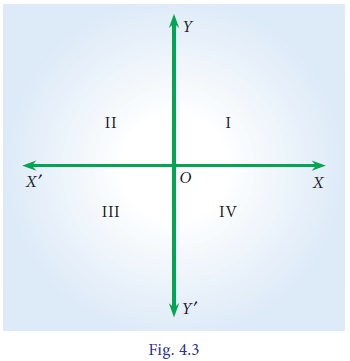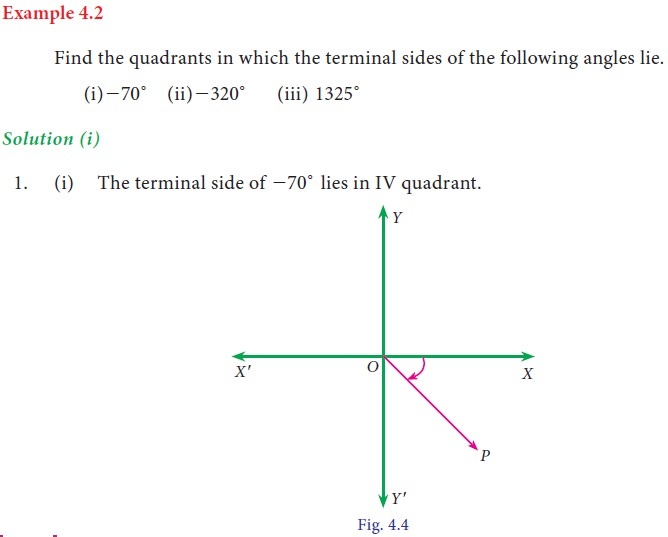Home | | Business Maths 11th std | Trigonometric ratios

# Trigonometric ratios

Using trigonometric ratios of the allied angles we can find the trigonometric ratios of any angle.

Trigonometric ratios

Let X' OX and Y' OY be two lines at right angles to each other as in the figure. We call X' OX and Y' OY as X axis and Y axis respectively. Clearly these axes divided the entire plane into four equal parts called “Quadrants” .

The parts XOY, YOX' , X'OY' and Y'OX are known as the first, second, third and the fourth quadrant respectively.## 2. Signs of the trigonometric ratios of an angle θ as it varies from 0º to 360º

In the first quadrant both x and y are positive. So all trigonometric ratios are positive. In the second quadrant (90° < θ  <180°) x is negative and y is positive. So trigonometric ratios sin θ and cosec θ are positive. In the third quadrant (180° < θ  < 270°) both x and y are negative. So trigonometric ratios tan θ and cot θ are positive. In the fourth quadrant (270° < θ < 360° ) x is positive and y is negative. So trigonometric ratios cos θ and sec θ are positive.## 3. Trigonometric ratios of allied angles:

Two angles are said to be allied angles when their sum or difference is either zero or a multiple of 90° . The angles -θ , 90° ± θ, 180° ± θ,, 360° ± θ etc .,are angles allied to the angle θ. Using trigonometric ratios of the allied angles we can find the trigonometric ratios of any angle.Tags : Definition, Formula, Solved Example Problems, Exercise | Mathematics , 11th Business Mathematics and Statistics(EMS) : Chapter 4 : Trigonometry
Study Material, Lecturing Notes, Assignment, Reference, Wiki description explanation, brief detail
11th Business Mathematics and Statistics(EMS) : Chapter 4 : Trigonometry : Trigonometric ratios | Definition, Formula, Solved Example Problems, Exercise | Mathematics